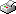Print This Page Bertil Wegmann -- doctoral thesis -- Bayesian Inference in Structural Second-Price Auctions Abstract The aim of this thesis is to develop efficient and practically useful Bayesian methods for statistical inference in structural second-price auctions. The models are applied to a carefully collected coin auction dataset with bids and auctionspecific characteristics from one thousand Internet auctions on eBay. Bidders are assumed to be risk-neutral and symmetric, and compete for a single object using the same game-theoretic strategy. A key contribution in the thesis is the derivation of very accurate approximations of the otherwise intractable equilibrium bid functions under different model assumptions. These easily computed and numerically stable approximations are shown to be crucial for statistical inference, where the inverse bid functions typically needs to be evaluated several million times. In the first paper, the approximate bid is a linear function of a bidder's signal and a Gaussian common value model is estimated. We find that the publicly available book value and the condition of the auctioned object are important determinants of bidders' valuations, while eBay's detailed seller information is essentially ignored by the bidders. In the second paper, the Gaussian model in the first paper is contrasted to a Gamma model that allows intrinsically non-negative common values. The Gaussian model performs slightly better than the Gamma model on the eBay data, which we attribute to an almost normal or at least symmetrical distribution of valuations. The third paper compares the model in the first paper to a directly comparable model for private values. We find many interesting empirical regularities between the models, but no strong and consistent evidence in favor of one model over the other. In the last paper, we consider auctions with both private-value and common-value bidders. The equilibrium bid function is given as the solution to an ordinary differential equation, from which we derive an approximate inverse bid as an explicit function of a given bid. The paper proposes an elaborate model where the probability of being a common value bidder is a function of covariates at the auction level. The model is estimated by a Metropolis-within-Gibbs algorithm and the results point strongly to an active influx of both private-value and commonvalue bidders. Key words: Asymmetry, Bid function approximation, Common values, Gamma model, Gaussian model, Markov Chain Monte Carlo, Private values, Variable selection, Internet auctions. ISBN 978-91-7447-276-9